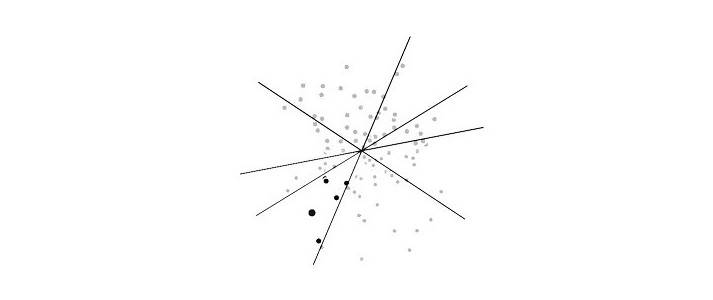# Locality-Sensitive Hashing# LSHashing (Locality-Sensitive Hashing)

Is an open source Python library under MIT License.LSHashing performs Locality-Sensitive Hashing to search for nearest neighbors in high dimensional data.

For now it only supports random projections but future versions will support more methods and techniques.

### Implementation

`pip install lshashing`

Make sure the data and query points are numpy arrays!.

```from lshashing import LSHRandom
import numpy as np

sample_data = np.random.randint(size = (20, 20), low = 0, high = 100)
point = np.random.randint(size = (1,20), low = 0, high = 100)

lshashing = LSHRandom(sample_data, hash_len = 4, num_tables = 2)

print(lshashing.tables.hash_table)
#{225: [0, 12],
# 121: [1, 2, 3, 4, 5, 7, 8, 9, 10, 13, 15, 16, 19],
# 81: [6, 11],
# 196: ,
# 100: [17, 18]}

print(lshashing.knn_search(sample_data, point, k = 4, buckets = 3, radius = 2))
#[Neighbor(index=7, distance=159.8217757378512, value=[[78 35 94]...]),
# Neighbor(index=13, distance=174.1551032843999, value=[[86 48 32]...]),
# Neighbor(index=19, distance=174.5737666432159, value=[[53 52 22]...]),
# Neighbor(index=16, distance=180.87564789103038, value=[[81 91 70]...])]```

lshashing also supports parallelism using joblib library.

```sample_data = np.random.randint(size = (20, 20), low = 0, high = 100)
point = np.random.randint(size = (1, 20), low = 0, high = 100)

lsh_random_parallel = LSHRandom(sample_data, 4, parallel = True)
lsh_random_parallel.knn_search(sample_data, point, 4, 3, parallel = True)
# [Neighbor(index=7, distance=137.0729732660673, value=[[76 16 41]...]),
#  Neighbor(index=1, distance=163.25133996387288, value=[[81 55 41]...]),
#  Neighbor(index=4, distance=172.41519654601214, value=[[33 21  0]...]),
#  Neighbor(index=8, distance=183.0327839486686, value=[[70 27 85]...])]```

Simply you can add new entries to the hash tables using the add_new_entry method.

```from lshashing import LSHRandom
import numpy as np

sample_data = np.random.randint(size = (15, 20), low = 0, high = 100)
point = np.random.randint(size = (1, 20), low = 0, high = 100)

lshashing = LSHRandom(sample_data, hash_len = 3, num_tables = 2)
print(lshashing.tables.hash_table)
# {4: [0, 1, 3, 6], 49: [2, 4, 8, 11, 14], 36: [5, 9, 12], 9: [7, 10, 13]}

print(lshashing.n_rows)
# 15

print(lshashing.n_rows)
# 16

print(lshashing.tables.hash_table)
# {4: [0, 1, 3, 6, 15], 49: [2, 4, 8, 11, 14], 36: [5, 9, 12], 9: [7, 10, 13]}```

Locality-sensitive hashing is an approximate nearest neighbors search technique which means that the resulted neighbors may not always be the exact nearest neighbor to the query point. To enhance and ensure better extactness, hash length used, number of hash tables and the buckets to search need to be tweaked.

I also made some comparison, the script is available in the github repo (see link above), between lshashing, linear method to get KNNs and scikit-learn’s BallTree and KDTree and here are the results.

```python examples/lshashing_compare.py

# lshashing module
# Sample data shape:  (20000, 15000)

# query point
# (15000,)

# Start comparison in searching for 4 NNs
# time to perform:  143.73057675361633

# ##### Search with lshashing package:
# time to construct lsh:  1.6940016746520996
# time to perform:  4.987746477127075

# ##### Search with lshashing package in parallel:
# time to construct lsh:  8.06048321723938
# time to perform:  4.106183767318726

# ##### Now with Scikit Learn
# time to construct ball_tree:  55.20065641403198
# time to perform:  0.43003249168395996

# ##### With sklearn KDTree
# time to construct the tree:  82.20907664299011
# time to perform:  0.49397754669189453

# ##### basic scikit-learn
# time to fit dataset:  90.42933940887451
# time to perform:  0.5779902935028076```